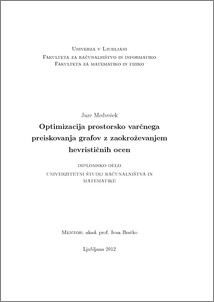# Optimization of space-saving graph search with approximate heuristic values

Jure Medvešek (2012) Optimization of space-saving graph search with approximate heuristic values. EngD thesis.Preview
PDF

## Abstract

Finding an optimal path in a state space is often very difficult in practise. The goal of this diploma thesis is to find a space efficient algorithm which finds an optimal solution within an acceptable time frame, even in large problem spaces. Algorithm A* is relatively fast, but it has exponential space complexity. Algorithms with linear space complexity can have quadratic time complexity, in terms of A*'s time complexity. In both cases we do not check for duplicates achieved by different paths. For this type of graphs we propose an optimization of the IDA* algorithm, where we granulate the heuristic function f. The new algorithm finds an optimal solution with time complexity O(N logN), where N is the number of nodes expanded by algorithm A*. Space complexity is linear in the length of the longest expanded path. The same idea can be applied to algorithm RBFS where RBFS can be even slightly faster than IDA*.

Item Type: Thesis (EngD thesis)
Keywords: search, optimization, granulation
Number of Pages: 32
Language of Content: Slovenian
Mentor / Comentors:
Name and SurnameIDFunction